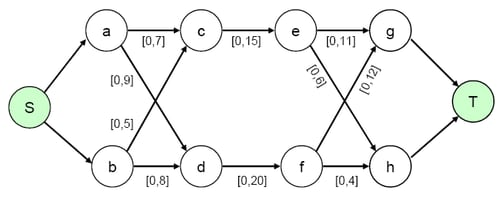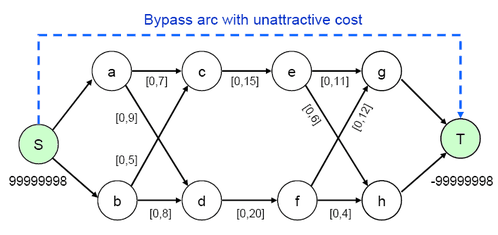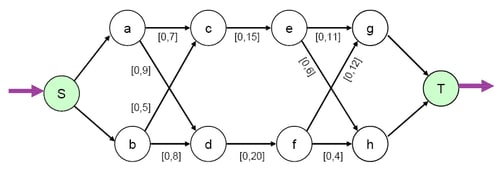### Example 6.10 Maximum Flow Problem

Consider the maximum flow problem depicted in Output 6.10.1. The maximum flow between nodes S and T is to be determined. The minimum arc flow and arc capacities are specified as lower and upper bounds in square brackets, respectively.

Output 6.10.1: Maximum Flow Problem ExampleYou can solve the problem using either EXCESS=ARCS or EXCESS=SLACKS. Consider using the EXCESS=ARCS option first. You can use the following SAS code to create the input data set:

```data arcs;
input _from_ \$ _to_ \$ _cost_ _capac_;
datalines;
S a  .  .
S b  .  .
a c  1  7
b c  2  9
a d  3  5
b d  4  8
c e  5 15
d f  6 20
e g  7 11
f g  8  6
e h  9 12
f h 10  4
g T  .  .
h T  .  .
;
```

You can use the following call to PROC NETFLOW to solve the problem:

```title1 'The NETFLOW Procedure';
proc netflow
intpoint
maxflow
excess = arcs
arcdata = arcs
source  = S    sink = T
conout  = gout3;
run;
```

With the EXCESS=ARCS option specified, the problem gets transformed internally to the one depicted in Output 6.10.2. Note that there is an additional arc from the source node to the sink node.

Output 6.10.2: Maximum Flow Problem, EXCESS=ARCS Option SpecifiedThe output SAS data set is displayed in Output 6.10.3.

Output 6.10.3: Maximum Flow Problem, EXCESS=ARCS Option Specified

 The NETFLOW Procedure

Obs _from_ _to_ _cost_ _capac_ _LO_ _SUPPLY_ _DEMAND_ _FLOW_ _FCOST_
1 g T 0 99999999 0 . 99999998 16.9996 0.0000
2 h T 0 99999999 0 . 99999998 8.0004 0.0000
3 S a 0 99999999 0 99999998 . 11.9951 0.0000
4 S b 0 99999999 0 99999998 . 13.0049 0.0000
5 a c 1 7 0 . . 6.9952 6.9952
6 b c 2 9 0 . . 8.0048 16.0097
7 a d 3 5 0 . . 4.9999 14.9998
8 b d 4 8 0 . . 5.0001 20.0002
9 c e 5 15 0 . . 15.0000 75.0000
10 d f 6 20 0 . . 10.0000 60.0000
11 e g 7 11 0 . . 10.9996 76.9975
12 f g 8 6 0 . . 6.0000 48.0000
13 e h 9 12 0 . . 4.0004 36.0032
14 f h 10 4 0 . . 4.0000 40.0000

You can solve the same maximum flow problem, but this time with EXCESS=SLACKS specified. The SAS code is as follows:

```title1 'The NETFLOW Procedure';
proc netflow
intpoint
excess  = slacks
arcdata = arcs
source  = S    sink = T
maxflow
conout  = gout3b;
run;
```

With the EXCESS=SLACKS option specified, the problem gets transformed internally to the one depicted in Output 6.10.4. Note that the source node and sink node each have a single-ended excess arc attached to them.

Output 6.10.4: Maximum Flow Problem, EXCESS=SLACKS Option SpecifiedThe solution, as displayed in Output 6.10.5, is the same as before. Note that the `_SUPPLY_` value of the source node Y has changed from 99999998 to missing S, and the `_DEMAND_`value of the sink node Z has changed from99999998 to missing D.

Output 6.10.5: Maximal Flow Problem

 The NETFLOW Procedure

Obs _from_ _to_ _cost_ _capac_ _LO_ _SUPPLY_ _DEMAND_ _FLOW_ _FCOST_
1 g T 0 99999999 0 . D 16.9993 0.0000
2 h T 0 99999999 0 . D 8.0007 0.0000
3 S a 0 99999999 0 S . 11.9867 0.0000
4 S b 0 99999999 0 S . 13.0133 0.0000
5 a c 1 7 0 . . 6.9868 6.9868
6 b c 2 9 0 . . 8.0132 16.0264
7 a d 3 5 0 . . 4.9999 14.9998
8 b d 4 8 0 . . 5.0001 20.0002
9 c e 5 15 0 . . 15.0000 75.0000
10 d f 6 20 0 . . 10.0000 60.0000
11 e g 7 11 0 . . 10.9993 76.9953
12 f g 8 6 0 . . 6.0000 48.0000
13 e h 9 12 0 . . 4.0007 36.0061
14 f h 10 4 0 . . 4.0000 40.0000